# Fowler's Conjecture on eigenvalues of (3,6)-polyhedra (Solved)

 Importance: Medium ✭✭
 Author(s): Fowler, Patrick W.
 Subject: Graph Theory » Algebraic Graph Theory
 Keywords: (3,6)-polyhedron eigenvalues
 Posted by: Robert Samal on: April 19th, 2007
 Solved by: M.DeVos,L.Goddyn,B.Mohar,R.Šámal: Cayley sum graphs and eigenvalues of $(3,6)$-fullerenes, Journal of Combinatorial Theory B 99 (2009), no.2, 358--369, doi:10.1016/j.jctb.2008.08.005
Conjecture   Letbe the graph of a-polyhedron with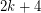vertices. Then the eigenvalues ofcan be partitioned into three classes:,(whereis nonnegative for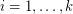), and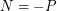.

A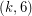-polyhedron is a cubic graph embedded in the plane so that all of its faces are-gons or hexagons. Such graphs exist only for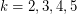. The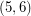-polyhedra are also known as fullerene graphs since they correspond to the molecular graphs of fullerenes.

The-polyhedra have precisely 4 triangular faces and they cover the complete graph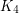. Therefore, the eigenvalues,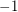,,ofare also eigenvalues of every-polyhedron. Patrick Fowler computed eigenvalues of numerous examples and observed that all other eigenvalues occur in pairs of opposite values,, a similar phenomenon as for bipartite graphs. From the spectral information, the-polyhedra therefore behave like a combination ofand a bipartite graph.

Horst Sachs and Peter John (private communication) found some reduction procedures which allow Fowler's Conjecture to be proved for many infinite classes of (3,6)-polyhedra.

## Bibliography

[FJS] P. W. Fowler, P. E. John, H. Sachs, (3,6)-cages, hexagonal toroidal cages, and their spectra, Discrete mathematical chemistry (New Brunswick, NJ, 1998), pp. 139-174, DIMACS Ser. Discrete Math. Theoret. Comput. Sci. 51, Amer. Math. Soc., Providence, RI, 2000. MathSciNet

[M] B. Mohar: Problem of the Month

* indicates original appearance(s) of problem.

### Is it done?

What is the status of your possible proof?

Gordon Royle

You can find the proof on the arxiv:

Matt DeVos, Luis Goddyn, Bojan Mohar, Robert Samal: Cayley sum graphs and eigenvalues of-fullerenes

Robert Samal

### Coming Soon

The proof is solid, but the paper is still in preliminary form.. coming soon!

### Solved!?

Together with Luis Goddyn, Bojan Mohar, and Robert Samal, we believe we have solved this conjecture in the affirmative. As soon as there is a paper in the ArXiv, we'll post a link to it here.

Matt DeVos